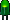• Home• Patreon• Links• Books
Number Converting:

# 2. Binary Number Conversion

(article continued from previous page)

## Binary to Octal

An easy way to convert from binary to octal is to group binary digits into sets of three, starting with the least significant (rightmost) digits.

 Binary: 11100101 = 11 100 101 011 100 101 Pad the most significant digits with zeros if necessary to complete a group of three.

Then, look up each group in a table:

 Binary: 000 001 010 011 100 101 110 111 Octal: 0 1 2 3 4 5 6 7

 Binary = 011 100 101 Octal  = 3 4 5 = 345 oct

An equally easy way to convert from binary to hexadecimal is to group binary digits into sets of four, starting with the least significant (rightmost) digits.

Binary: 11100101 = 1110 0101

Then, look up each group in a table:

 Binary: 0000 0001 0010 0011 0100 0101 0110 0111 Hexadecimal: 0 1 2 3 4 5 6 7
 Binary: 1000 1001 1010 1011 1100 1101 1110 1111 Hexadecimal: 8 9 A B C D E F

 Binary = 1110 0101 Hexadecimal = E 5 = E5 hex

## Binary to Decimal

They say there are only 10 people in this world: those that understand binary and those that don’t. Ha ha.

If you don’t get that joke, you'll need a method to convert from binary to decimal. One method involves addition and multiplication.

1. Start the decimal result at 0.
2. Remove the most significant binary digit (leftmost) and add it to the result.
3. If all binary digits have been removed, you’re done. Stop.
4. Otherwise, multiply the result by 2.
5. Go to step 2.

Here is an example of converting 11100000000 binary to decimal:

 Binary Digits Operation Decimal Result Operation Decimal Result 11100000000 +1 1 × 2 2 1100000000 +1 3 × 2 6 100000000 +1 7 × 2 14 00000000 +0 14 × 2 28 0000000 +0 28 × 2 56 000000 +0 56 × 2 112 00000 +0 112 × 2 224 0000 +0 224 × 2 448 000 +0 448 × 2 896 00 +0 896 × 2 1792 0 +0 1792 done.

Let’s try converting from decimal...